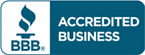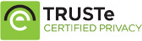Sale!

# Solution manual for Statistics for Business and Economics 8th Edition Betty Newbold

\$57.00 \$30.00

Statistics for Business and Economics 8th Edition Betty Newbold Solutions to end of chapter questions

Full chapters are included

You want Test bank?## Description

Solution manual for Statistics for Business and Economics 8th Edition Betty Newbold

Table of content

Chapter 1: Describing Data: Graphical
Chapter 2: Describing Data: Numerical
Chapter 3: Probability
Chapter 4: Discrete Random Variables and Probability Distributions
Chapter 5: Continuous Random Variables and Probability Distributions
Chapter 6: Sampling and Sampling Distributions
Chapter 7: Estimation: Single Population
Chapter 8: Estimation: Additional Topics
Chapter 9: Hypothesis Testing: Single Population
Chapter 10: Hypothesis Testing: Additional Topics
Chapter 11: Simple Regression
Chapter 12: Multiple Regression
Chapter 13: Additional Topics in Regression Analysis
Chapter 14: Analysis of Categorical Data
Chapter 15: Analysis of Variance
Chapter 16: Time-Series Analysis and Forecasting
Chapter 17: Additional Topics in Sampling